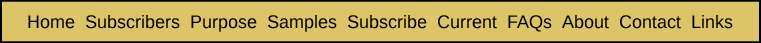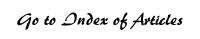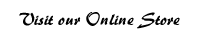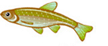There is No Comparison This article provides an update to a simple cluster rating system first presented in the July 2002 Bible Code Digest, and is the continuation of an article that appeared in our July/August 2005 Digest. The code examples presented by skeptics in no way compare to the clusters and matrices discovered by some code researchers. There is a tremendous gap between the two. To get a clear sense of the magnitude of this gap, we present the results of applying a simple, yet reasonably accurate, approach to comparing different code matrices and clusters in terms of their improbability. The results, without naming names, are quite revealing: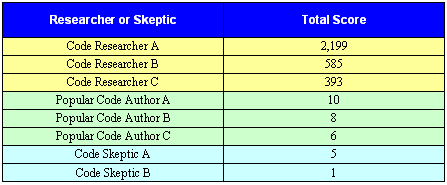The total score shown above is the sum of the total score of the two most extensive code findings presented by each researcher, author or skeptic. In each cluster, every code with eight or fewer letters does not count. For every code with eight or more letters, we subtract eight from the number of letters in the code, then the results are added together for the total score. Example formula for a cluster containing three codes each 8 or more letters. Each code length (a, b, and c) is subtracted by 8, then the results are added together for the total score. (a-8) + (b-8) + (c-8) = Total Score For a cluster with three codes of 8, 9, and 10 letters respectively, the formula would read: (8-8) + (9-8) + (10-8) = 0 + 1 + 2 = 3 Clusters with more lengthy codes have a higher score. The higher the score, the more improbable the cluster is. For a cluster with three codes of 20, 25, and 30 letters respectively, the formula would read: (20-8) + (25-8) + (30-8) = 12 + 17 + 22 = 51 A cluster with the score of 51 is far more improbable than a cluster with a score of 3. Another way of showing the formula is to add up all the letters of the codes of length 8 or more. Then subtract 8 times the total number of codes with 8 or more letters. Where A is the total number of letters of the codes with a length of 8 or more and B is the total number of codes with a length of 8 or more: A � (8 x B) = Total Score So, the formula for a cluster of three codes of eight or more letters, with a total of 30 letters would read: 30 � (8 x 3) = 6 So, how do the different code findings rate by this simple system? The tables presented in this article provide the results. First, we will look at the clusters offered by Brendan McKay, a Bible code skeptic, on his Scientific Refutation of the Bible Codes website. The following table shows the results of the Hanukah cluster from War and Peace and the Moby Dick assassination codes.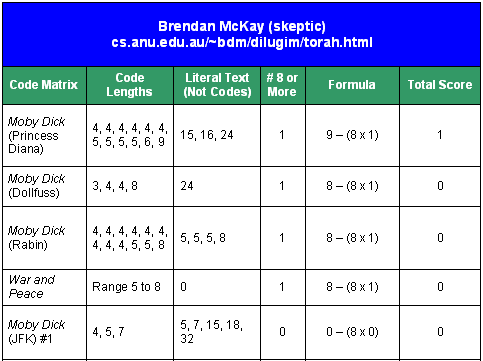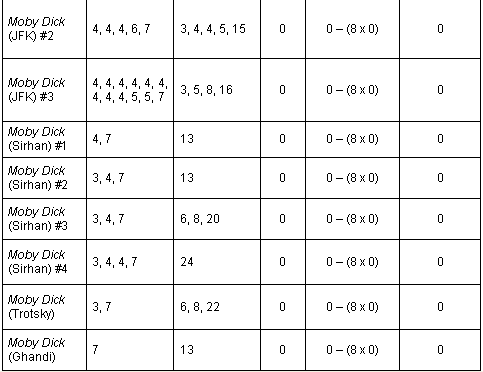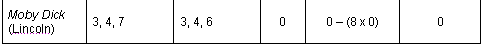In the above chart we have noted the lengths of the sections of literal text that were made to appear to be "codes" in McKay's examples. He continued Drosnin's practice of marking literal text in this way in order to visually beef up the supposed substance of the "code" examples he was presenting. The next table shows the ratings of Dave Thomas's most extensive example clusters.Note: D.T. Hammerman is a "name" that Dave Thomas made up to describe himself. Please do not construe it as a legitimate name that Thomas looked for. Given that it is not a legitimate name, it is, therefore, a stretch to give this "code" three points. Now, we will look at the top-five-rated code clusters from each of Michael Drosnin's books.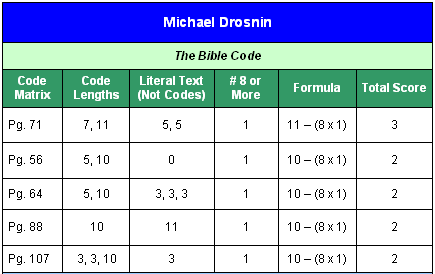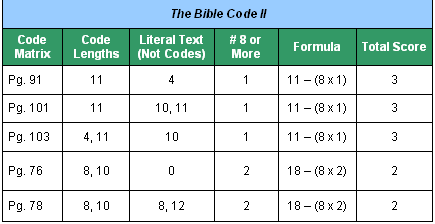Sadly, in The Bible Code, approximately 76% of the matrices had a score of zero, and in The Bible Code II, approximately 86% of the matrices had a score of zero. The trivial nature of Drosnin's examples has done much to discredit the phenomena of Bible codes in the minds of many. To read reviews of The Bible Code II, click here. In spite of the severe shortcomings in Drosnin's books, we still offer them in our online store. We do so for the reason that his books have had an important impact on the reputation of the field of Bible code research. Continue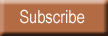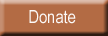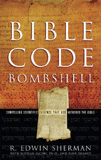Bombshell examines two massive, recently discovered clusters of codes in the Hebrew Old Testament. To read more about Bombshell, click here, or click below to order from Amazon today!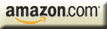| HOME | SUBSCRIBERS ONLY | OUR PURPOSE | FREE SAMPLES | CURRENT | | FAQs | ABOUT BIBLE CODES | SUBSCRIBE | CONTACT | Copyright © 2016 BibleCodeDigest.com